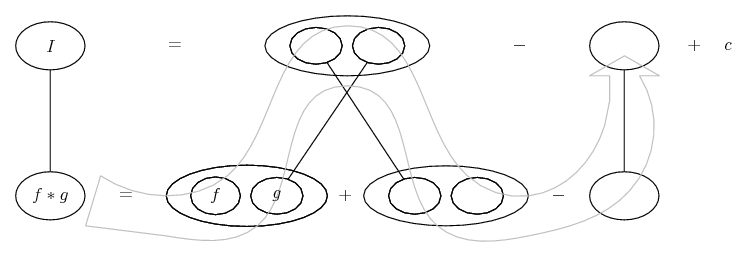# Thread: Stuck on Integration by Parts

1. ## Stuck on Integration by Parts

Question 1
$\displaystyle \int e^\frac1x x^2$
First thing I did was attempt integration by parts
$\displaystyle u=\frac 1x \ du=\frac 1{x^2} \ dv=x^2 \ v=\frac {x^3}3$

Using the formula
$\displaystyle UV-\int VdU$

I come up with
$\displaystyle (\frac 1x) (\frac13 x^3) - \frac13 \int \frac{x^3}{x^2}$

Here is where I get stuck with the integration, I think I can move the
$\displaystyle x^2$
up top and make it
$\displaystyle x^-2$

So I am left with
$\displaystyle \int {x^3}{x^-2}$
Above is Supposed to be a negative 2, but couldnt find the code for it.

So if I use u sub
$\displaystyle U=x^3 \ dU=3x^2$

The 3 I can handle but not sure what to do about the fact that my exponent is negative, and my dU has a positive.

Any help?

Thanks
Rich

2.Originally Posted by SpoolxQuestion 1
$\displaystyle \int e^\frac1x x^2$
First thing I did was attempt integration by parts
$\displaystyle u=\frac 1x \ du=\frac 1{x^2} \ dv=x^2 \ v=\frac {x^3}3$

Using the formula
$\displaystyle UV-\int VdU$

I come up with
$\displaystyle (\frac 1x) (\frac13 x^3) - \frac13 \int \frac{x^3}{x^2}$

Here is where I get stuck with the integration, I think I can move the
$\displaystyle x^2$
up top and make it
$\displaystyle x^-2$

So I am left with
$\displaystyle \int {x^3}{x^-2}$
Above is Supposed to be a negative 2, but couldnt find the code for it.

So if I use u sub
$\displaystyle U=x^3 \ dU=3x^2$

The 3 I can handle but not sure what to do about the fact that my exponent is negative, and my dU has a positive.

Any help?

Thanks
Rich
Dear Spoolx,

This integration could not be found using elementary functions. See Wolfram Mathematica Online Integrator

3. Thanks for the reply, I will do some more reading in the book, however its in the integration by parts section, so I figured it could be done that way.

How would you recommend solving this problem if I was given it on an exam?

4.Originally Posted by SpoolxThanks for the reply, I will do some more reading in the book, however its in the integration by parts section, so I figured it could be done that way.

How would you recommend solving this problem if I was given it on an exam?
I think there typo in the book. Integration by parts won't work. The maximum you could progress through integration by parts method is,

$\displaystyle \displaystyle\int x^2 e^{\frac{1}{x}}dx=\frac{x^3}{3}e^{\frac{1}{x}}+\fr ac{1}{6}x^2 e^{\frac{1}{x}}+\frac{1}{6}\int e^{\frac{1}{x}}dx$

Then to evaluate, $\displaystyle \int e^{\frac{1}{x}}dx$ you will need to know about the "Exponential integral". So I hope this will not be given in a exam at your level.5. But... parts once more takes you to

$\displaystyle \frac{1}{6} \int \frac{1}{x} e^{\frac{1}{x}} dx$

which is the ExpIntegralEi - Wolfram Mathematica 8 Documentation the exponential integral of 1/x.

http://www.wolframalpha.com/input/?i...281%2Fx%29%2Fx

So you can use parts to arrive at Wolfram's result, even though the special function is switching off the 'show steps' option that Wolfram usually offers.

Just in case a picture helps...... where (key in spoiler) ...

Spoiler:... is the product rule. Straight continuous lines differentiate downwards (integrate up) with respect to x. And,... is lazy integration by parts, doing without u and v.

(But, oh dear, the new MHF page layout isn't friendly to wide diagrams.)_________________________________________

Don't integrate - balloontegrate!

Balloon Calculus; standard integrals, derivatives and methods

Balloon Calculus Drawing with LaTeX and Asymptote!

6.Originally Posted by tom@ballooncalculusBut... parts once more takes you to

$\displaystyle \frac{1}{6} \int \frac{1}{x} e^{\frac{1}{x}} dx$

which is the ExpIntegralEi - Wolfram Mathematica 8 Documentation

So you can use parts to arrive at Wolfram's result, even though the special function is switching off the 'show steps' option that Wolfram usually offers.
Hey! Thats a suprise. The exponential integral is defined as $\displaystyle Ei(x)=\displaystyle\int{\frac{e^{\frac{1}{x}}}{x}} dx$. Therefore you are correct. The answer could be obtained easily. And thank you very much for pointing that, I learnt something new.To Spoolx,

Sorry if I confused you in my previous post.When I saw the Ei abbreviation in wolfram thought it was something which we cant obtain. The problem is correct.

7.Originally Posted by SpoolxQuestion 1
$\displaystyle \int e^\frac1x x^2$
First thing I did was attempt integration by parts
$\displaystyle u=\frac 1x \ du=\frac 1{x^2} \ dv=x^2 \ v=\frac {x^3}3$
Well, this is incorrect. You use 'integration by parts' to integrate $\displaystyle \int udv$ which, with this u and dv, would be $\displaystyle \int \frac{x^2}{x}dx= \int x dx$. What you have is $\displaystyle \int e^u dv$ and the integration by parts formula does not apply.

Using the formula
$\displaystyle UV-\int VdU$

I come up with
$\displaystyle (\frac 1x) (\frac13 x^3) - \frac13 \int \frac{x^3}{x^2}$

Here is where I get stuck with the integration, I think I can move the
$\displaystyle x^2$
up top and make it
$\displaystyle x^-2$

So I am left with
$\displaystyle \int {x^3}{x^-2}$
Above is Supposed to be a negative 2, but couldnt find the code for it.

So if I use u sub
$\displaystyle U=x^3 \ dU=3x^2$

The 3 I can handle but not sure what to do about the fact that my exponent is negative, and my dU has a positive.

Any help?

Thanks
Rich

#### Search Tags

integration, parts, stuck